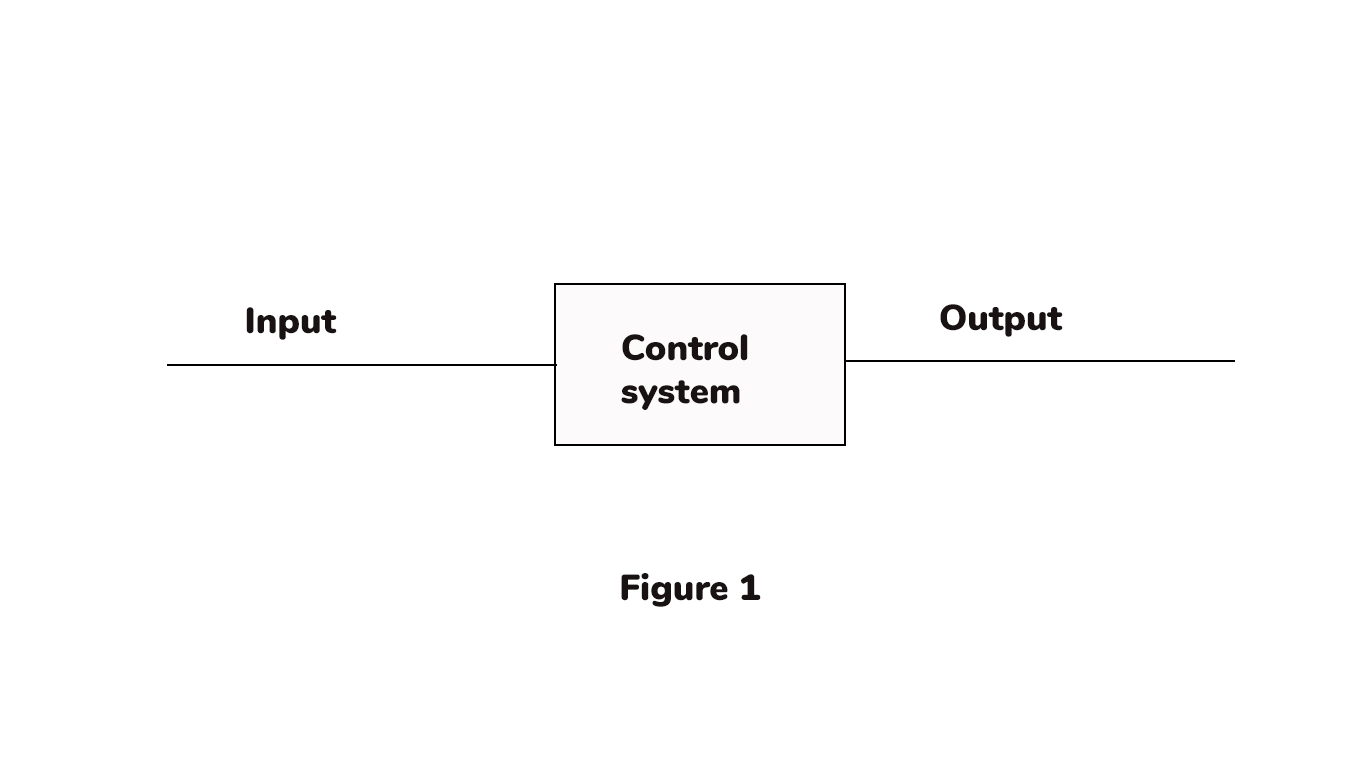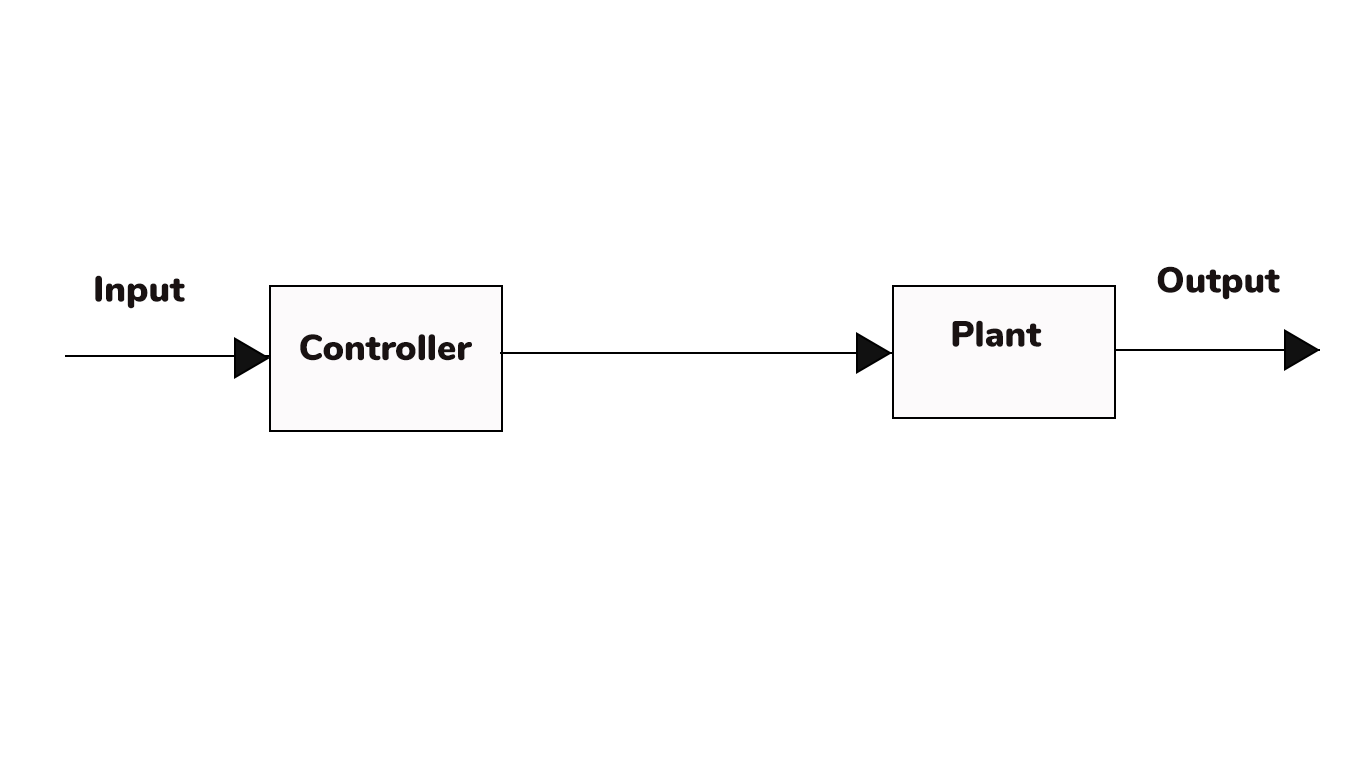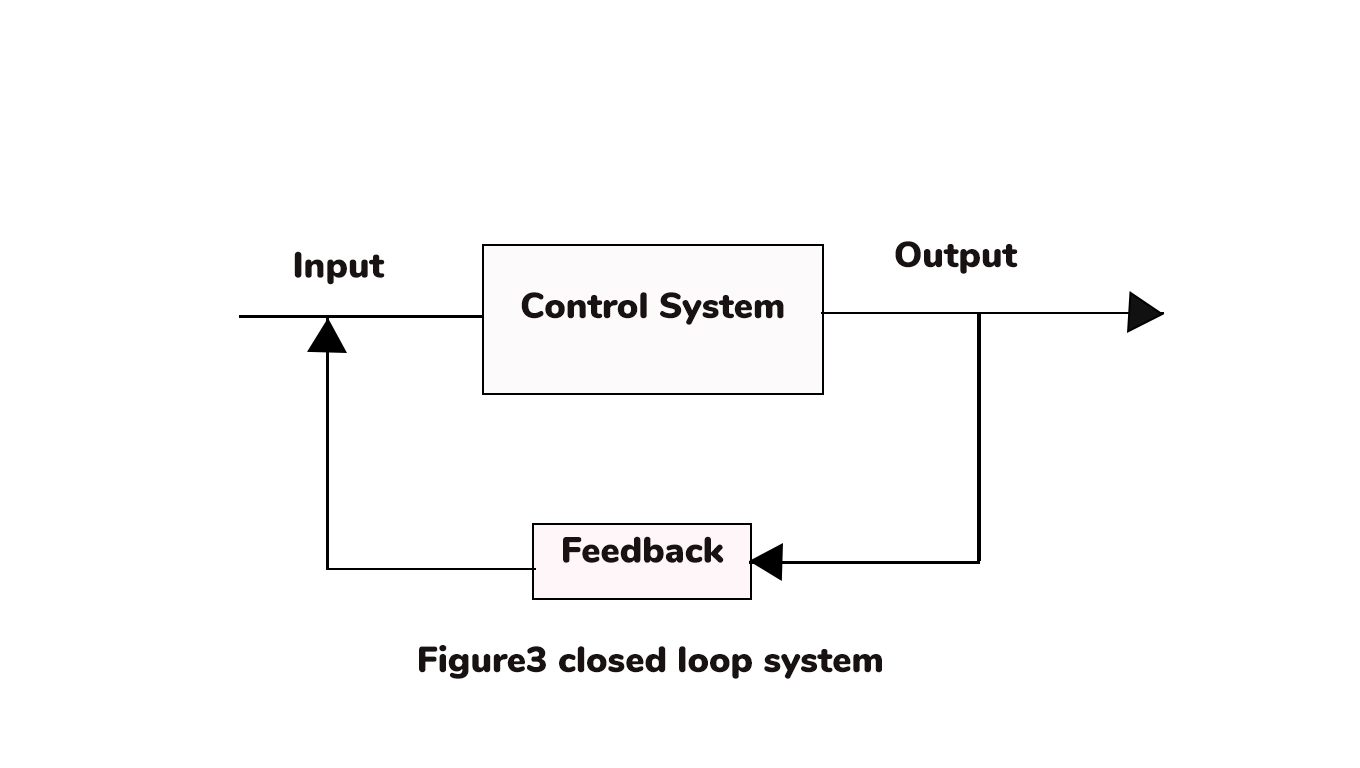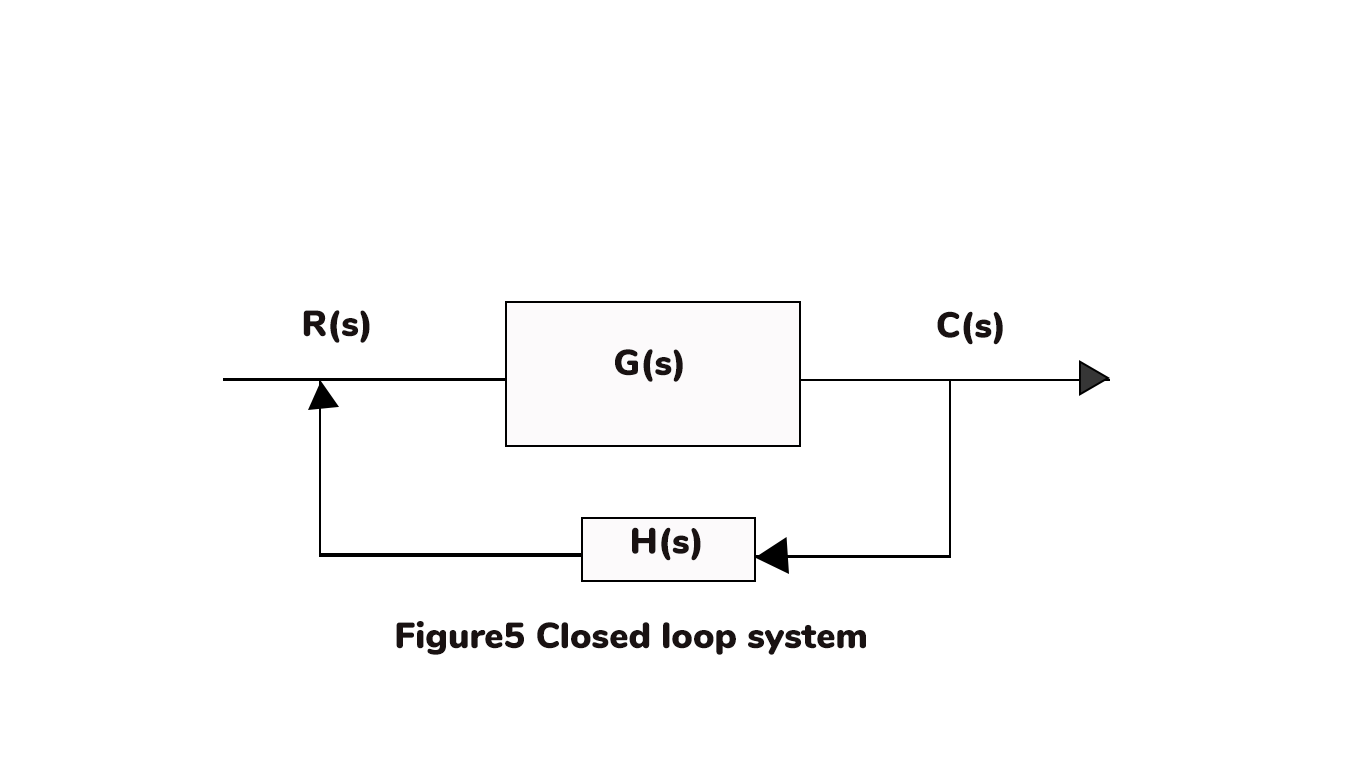### Loop system

Loop system :

Control systems play a major role in the engineering and day to life systems. The control of the system may depend on the requirement of our desired application. Basically it is divided into open loop control systems and closed loop control systems. The representation of a control system is shown in figure 1.For a control system the major elements are input and output. Based on the desired output the system will change to an open loop or closed loop control system.

Open loop system:

Open loop system the input  of the system never depends on the output. It simply says that the input is independent from the output. It simply represented as,Figure2 open loop system

Example, when traffic control is considered the vehicles will be moved when a signal is green displayed and the vehicle will stop when a red signal appears. So it simply shows the open loop control system. That is the input of the system works independent of output.

Closed loop system:

closed loop system the input  of the system depends on the output. It simply says that the input is dependent on the output. It simply represented as,Example, when traffic control is considered according to the density of vehicles  then the sensor senses the density of the vehicles and allows them to move. So it simply shows the closed loop control system. It shows it depends on the feedback of the output. That is the input of the system depends on the output.

Difference between open loop and closed loop system:-

 Open loop system Closed loop system It may not depend on feedback It depends on feedback It independent of output It depend on output The system is simple in design The system is difficult to design It is reliable It is not reliable In this the system is more stable In this the system is less stable In this may not get accurate calibration In this due to feedback calibration is accurate There is no possibility of optimization There is a possibility of optimization Less maintenance Difficult maintenance The response is slow The response is faster

Transfer function:-

The response of the output to the input is defined as a transfer function. Let's consider an open loop system as shown in figure 4.

Control systems play a major role in the engineering and day to life systems. The control of the system may depend on the requirement of our desired application. Basically it is divided into open loop control systems and closed loop control systems. The representation of a control system is shown in figure 1.For a control system the major elements are input and output. Based on the desired output the system will change to an open loop or closed loop control system.

Open loop system:

Open loop system the input  of the system never depends on the output. It simply says that the input is independent from the output. It simply represented as,Figure2 open loop system

Example, when traffic control is considered the vehicles will be moved when a signal is green displayed and the vehicle will stop when a red signal appears. So it simply shows the open loop control system. That is the input of the system works independent of output.

Closed loop system:

closed loop system the input  of the system depends on the output. It simply says that the input is dependent on the output. It simply represented as,Example, when traffic control is considered according to the density of vehicles  then the sensor senses the density of the vehicles and allows them to move. So it simply shows the closed loop control system. It shows it depends on the feedback of the output. That is the input of the system depends on the output.

Difference between open loop and closed loop system:-

 Open loop system Closed loop system It may not depend on feedback It depends on feedback It independent of output It depend on output The system is simple in design The system is difficult to design It is reliable It is not reliable In this the system is more stable In this the system is less stable In this may not get accurate calibration In this due to feedback calibration is accurate There is no possibility of optimization There is a possibility of optimization Less maintenance Difficult maintenance The response is slow The response is faster

Transfer function:-

The response of the output to the input is defined as a transfer function. Let's consider an open loop system as shown in figure 4.

The transfer function T(s)=C(s)R(s)= G(s)

Let’s consider a closed loop system as shown in figure 5.The transfer function.

T(s)=C(s)R(s)=G(s)1+G(s)H(s)

Properties of transfer function:

1. The transfer function should be a linear time-invariant system.
2. The transfer function does not depend on the input and output.
3. The transfer function is not applied to the system which has the initial values.
4. The transfer function depends on the feedback based on the system.

Procedure for a transfer function:

1. For a given system write a differential equation.
2. Assume all the initial conditions are zero.
3. Convert the differential equations to laplace transfer in step 1.
4. Identify the output and input of the system
5. Take the ratio of output to input as a transfer function.

Poles and zeros of transfer function:

The poles of the transfer function are those values of ‘s’ which makes the transfer function tend to infinity.

The zeros of the transfer function are those values of ‘s’ which makes the transfer function tend to zero.

Let us consider an example for transfer function=5(s+1)(s+2)/(s+3)(s+4)2

The roots for ‘s’ in the denominator are poles i.e s= -3,-4,-4.

The roots for ‘s’ in the numerator are zeros i.e s= -1,-2.

The transfer function T(s)=C(s)R(s)= G(s)

Let’s consider a closed loop system as shown in figure 5.The transfer function.

T(s)=C(s)R(s)=G(s)1+G(s)H(s)

Properties of transfer function:

1. The transfer function should be a linear time-invariant system.
2. The transfer function does not depend on the input and output.
3. The transfer function is not applied to the system which has the initial values.
4. The transfer function depends on the feedback based on the system.

Procedure for a transfer function:

1. For a given system write a differential equation.
2. Assume all the initial conditions are zero.
3. Convert the differential equations to laplace transfer in step 1.
4. Identify the output and input of the system
5. Take the ratio of output to input as a transfer function.

Poles and zeros of transfer function:

The poles of the transfer function are those values of ‘s’ which makes the transfer function tend to infinity.

The zeros of the transfer function are those values of ‘s’ which makes the transfer function tend to zero.

Let us consider an example for transfer function=5(s+1)(s+2)/(s+3)(s+4)2

The roots for ‘s’ in the denominator are poles i.e s= -3,-4,-4.

The roots for ‘s’ in the numerator are zeros i.e s= -1,-2.